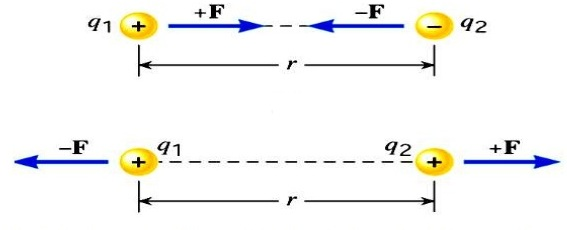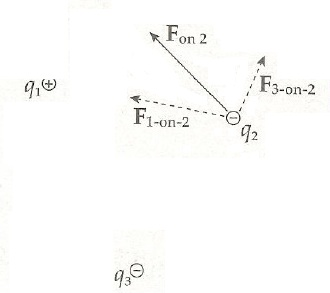# Coulomb’s law Definition Equation & Formula

This page is dedicated for providing best and up to date information about the coulomb’s law. In addition you can find the definition, equation and formula for understanding the basic concept of coulomb’s law.

You already know that when two charged bodies come closer there is always a force of attraction or force of repulsion. What is the behavior of that electron force and what is its magnitude this was experimentally established by French scientist Charles Coulomb (1736– 1806) in 1785.

This is now the fundamental law of electric force between two stationary charged particles and famous as coulomb’s law of electric forces.

Torsion Balance & Coulomb’s Law

Charles Augustin de Coulomb created a device that helped him develop his theories on charges and electric force and field. This device was invention of Coulomb to measure very weak force as well. The device name is Torsion Balance, which contributed in the creation of Coulomb’s Law by measuring Coulomb’s Constant.

Also same device was used by Henry Cavendish to find the gravitational constant. When an unknown force is applied to the metallic object or plates in the container, the object spins. The greater the force is, the bigger the angle.

## Coulomb’s Law Definition

This law states that the force of attraction or repulsion between two point charges is directly proportional to the product of the magnitude of charges and inversely proportional to the square of the distance between them.

Since there are two parts of the definition therefore we shall get two equations:

F ∝ q1 q2   ….. equation 1 basis is the electrostatic force directly proportional to product of charges.

F ∝ 1/r2   ….. equation 2 basis is electrostatic force inversely proportional to distance of charges.

combining above two equations, removing the proportionality sign, and adding proportionality constant gives us the below equation which is Coulomb’s law equation or formula.

F = k q1 q2 / r2 Newtons  …….. Coulombs law equationhere F is the force between the two charges and is called the Coulomb force and unit is Newton,

q1 and q2 are the magnitudes of two charges and ‘r’ is the distance between the two charges.

k is the constant of proportionality or coulomb’s constant. The value of k depends upon the medium between the two charges. If the medium between the two charges is air, then the value of k in SI units will be 𝟗 × 𝟏𝟎𝟗 𝑵𝒎𝟐 𝑪−𝟐 .

Coulomb’s constant can further be explained as below:

K= 1/4πεo

εo = 8.84 x 10-12  C2 / Nm2

### Vector of Coulomb’s Law

Electric force is a vector force. The first vector value is the sign of force, since the force can be either negative or positive. The second vector value is the angle and direction to which the force heads. for example in below picture the resulting force is F on 2, since q2 is repelled away by q3 and attracted to q1, because of charge movements.#### Important notes about Coulomb’s Law

1. Coulomb’s law is true only for point charges whose sizes are very small as compared to the distance between them.
2. Coulomb’s law is fully accurate only when the objects are stationary, and remains approximately correct only for slow movement. These conditions are collectively known as the electrostatic approximation.
3. Coulomb’s Constant being one of the constants with biggest numbers, Coulomb’s Constant shows that electromagnetic force is one of the strongest forces in the universe.
error: Content is protected !!